# Mild Hybrids....

Discussion in 'Hybriding' started by Frustrated Palm, Dec 25, 2002.

1. ### Frustrated Palm

Messages:
4,473
Anyone into Mild Hybrids. Hybrids that are 'game legal'. As in they dont break all the rules of physics and stuff. EX. RSX with Mid Engine and S4 turbo. Not that Macho 50000HP, 40 lbs, 0-60 in .001 seconds stuff. If you have any mild hybrids post them here! I will post some later.....

2. ### skynyrd

Messages:
838
Ah yes, now that's my favorite kind. I've got nothing against those huge hp hybrids, because they are fun to drive, but the more realistic ones (heh) are usually more enjoyable. Like the PT Cruiser I made in GT3 that has the Viper Engine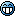Or Hemi Charger, challenger, baracuda, etc. I made in GT2.

3. ### Satty97

Messages:
18
Civic Type R ZZII AWD Skyline engine

[GT3CarData]
Region=US
BaseType= spoon_civic_00
BaseName= Spoon Civic Type-R(J)
0=-998626344
4=-1606925607
8=-834
12=-1
16=-1
20=-1
24=-1963651533
28=1555198238
32=-1963651020
36=1681027358
40=-1963651212
44=565342494
48=-1937302963
52=-1847534766
56=158873316
60=-1609951638
64=-1963651344
68=590508318
72=-1963652243
76=456290590
80=-1963651987
84=-600674018
88=-1963651957
92=1538421022
96=-1963652344
100=1538421022
104=-2619186
108=-1
112=-1
116=-1
120=-1963651856
124=1597141278
128=-1
132=-1
136=-1963651085
140=-1531809506
144=-1
148=-1
152=-1963651436
156=506622238
160=-1963651984
164=1538421022
168=-3405666
172=-1
176=-1963651855
180=1513255198
184=-1963651439
188=531788062
192=-1
196=-1
200=-1963652017
204=-1691193058
208=-1
212=-1
216=-12514
220=-1
224=-12514
228=-1
232=-101494757
236=1292648516
240=-2691
244=-2711
248=-1827
252=-1334
256=-1022
260=-821
264=-692
268=-16236
272=-4401
276=-32
280=-34
284=-10
288=-10
292=-20
296=-27
300=-1
304=-1
308=-1
312=-1
316=-1
320=-1
324=-21
328=-11
332=-84
336=-86
340=-129
344=-129
348=-158
352=-151
356=-76
360=-76
364=-8
368=-8
372=-8
376=-8
380=-8
384=-8
388=-8
392=-8
396=-7
400=-7
404=-11
408=-1
412=-41
416=-1
420=-21
424=-1
428=-4401
432=-10
436=-1
440=-1
444=-2
448=-2
452=-2
456=-2
460=-1
464=-1145989072
468=-24188357
472=-2639882
476=-16578
480=-1191296115
484=-1191296115
488=-1059792705
492=-1
496=-1
500=-1
504=-1
508=-2
512=-43

4. ### Frustrated Palm

Messages:
4,473
Acura RSX MR.

Just give it an S4 turbo, the MR drivetrain from the Jaguar Road Car, and then give it all of it own upgrades (except NA tune) from the tune shop. Handles great! I use it often!

Frustrated Palm Tree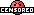5. ### Frustrated Palm

Messages:
4,473
BTW, I am also working on a whole RSX line of mild hybrids. Rally cars, drag cars (both ff and fr), and am trying to make an LM car (just no fancy looks).

6. ### F20

Messages:
108
dude, why don't u try a RSX/NSX hybrid??

7. ### Frustrated Palm

Messages:
4,473
Did already. Made RSX an FR and did engine swap among other things.....

8. ### IDAFC21

Messages:
1,596
I love mild hybrids, id rather drive one of those than one that just blows the crap out of everything. Lets see if i can remember some of my favorites....Ive got an RSX one, made it RWD, put ruf 3400 tires on it, threw a pt cruiser engine in it (just cuz i like the way it sounds) and bumped it up to about 500HP or so. wont beat any of the JGTC cars but its fun to drive.

Put the lancer rally engine in an FTO and made it 4wd, has about 500hp too, that cars pretty quick. My favorite one is a 240SX that i put an S15 engine into, r34 tires, Razo silvia chassis, f1 brakes, bumped it up to about 800hp, thats a nice car to drive. My second favorite is a 350Z w/ an R34 engine w/ its normal 1000hp, 4wd, skyline race car tires, f1 brakes...that thing HAULS, 8.8 second quarter i think and sticks to the road like glue

9. ### Frustrated Palm

Messages:
4,473
Acura RSX with a Toyota Denso Sard Supra Engine, 4WD, C5 exhaust (to give better sound), F1 brakes. Geez that car sounds great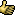and is fast too. 510 HP...

10. ### Frustrated Palm

Messages:
4,473
BTW, welcome to GTPlanet...

11. ### TingBoy

Messages:
1,182
i made a Lotus Elise wit a Spoon S2000 engine, spoon engine tune-ups, and the rest are lotus elise stuff.
This is sorta based on a car someone actually made at the LA auto show. ^_^ He put a B16C (Acura Integra Type R engine) in a lotus elise and it now has a 0-60 time equal to a ferrari F-50.
of couse i used a different engine ^_^ cuz he said that he was gonna put a K20A (Japanese Integra Type R) and a F20C(S-2000) in his next Elises.

12. ### Frustrated Palm

Messages:
4,473
Yeah the Lotus is a alight car. Practically NO welds. Most of the frame work is held by a superstong adhesive which is lighter than welding...

13. ### Race Idiot(Banned)

Messages:
11,439
Bah why bother do that, when you can just put the old 2.2 turbo 4cyl unit that they used to do in the Esprit. I'm sure Lotus actually do this.

14. ### IDAFC21

Messages:
1,596
ThanksYou want a fun Elise...throw a suped up 1000hp Esprit engine in it, make it 4wd, and throw some larger tires on it. cant remember what i used, s2000 tires i think....WOW...does an 8.X somthin quarter and is a real trick to drive. its so damn light and so damn powerfull that if you go into a turn too fast you just slide right off the road...haha. Whats really fun to do in that one tho is turn the TCS off and go and do donutsit does some CRAZY donuts....especially if you do reverse donuts...i got dizzy...hehe

15. ### TingBoy

Messages:
1,182
nah, i don't like havin too much power ^_^

16. ### 12sec. Civic

Messages:
1,695
Here is my best mild Hybrid so far.

I took a Skyline GT-R M-Spec. I put in Calsonic Skyline chassis, brakes, drivetrain and LSD.

It was FR and it used the stock engine and tranny. It also has the Calsonic suspension.

It's engine is only modifiable to 445hp, but with a curb weight of 935kg (2,060lbs) it feels very fast.

I made a friend guess how much hp it had because he didnt know what I had done to it. He guessed 700hp.

I did a 4'07,600 on Complex String.

I think my Midfield time was around 1'05,800 and thats for a car with 445hp and is easier to drive than an F1. Its grip is way out there.

PS: At first I put on Calsonic Skyline T8 tires. And for everyones info, putting on another cars tires gives you the donor cars track(distance from left to right tire). The Calsonic Skyline actually has a wider stance than the regular Skyline's, so the tires stuck out. I just went and bought regular R34 T8's and it looks normal again.

17. ### Frustrated Palm

Messages:
4,473
Actually the chassis does that. Its just the Calsonic Skylines' tires are so wide...

18. ### Heydu

Messages:
908
Actually, neither one affects the track. FP is right about the width of the tires making it appear that the track is changed. The cars dimensions are set by these factors.

Chassis affects Weight, height, length and wheelbase.

Body/DF affects width, front track and rear track.

I can't say definately that all of these changes are visable to the naked eye, but if U check in the car specs in the garage u will see that the values change as a result of these mods.

19. ### HKS_KiD

Messages:
53
Is hybriding easy or do u have to know about gear ratios and things such as that?, I dont know about that stuff, i know about the chassis, curb weight, and stuff like that. Do u guys take transmissions of like a Denso Supra and use the same gear ratios or does it depend on what engine you use??

(P.S. A B16 is a JDM civic engine and a B18 is an JDM Integra engine, just lettn ya know)

20. ### 12sec. Civic

Messages:
1,695

Well, I just assumed this because if you check the Calsonic's track against the R34's track. The Calsonics is much wider.

And using the same wider Calsonic chassis, I bought R34 T8's and the tires dont stick out at all. So, it clearly wasnt the Calsonics chassis that caused the tires to stick out. But just the fact that the Calsonics Tires are actually wider them selves. Not to mention they are about 3 inches bigger in diameter as they stuck out above the hood by quite a bit.

I also intend on making one of these "Special Edition" cars using JGTC parts for a Supra and an NSX.

21. ### LowTEC-Derbo

Messages:
63
I love mild hybrids..more or so like real lift possible hybriding.

I have a few rice rocket inspired hondas. Civic EG6 with a B18c5 and Del sol with H22a (euro r edition), and Integra Type R with K20A.
-I've also done that elise with B18C5.
-Lexus IS turned into Lexus IS300 with Supra SZ-R engine (which is true to real life specs roughly..same engine code 2JZ-GE)
-Nissan Z Concept with RB26DETT R34 VSpec II edition.
-Audi TT with S4 Engine..(I believe there will be a v6 option for TT soon this year)
-Bmw 328ci with V8 Vantage..(sounds like a BMW now)
-Opel Speedster with GT40 Race Car Engine
-RX7 with 2JZ-GTE (saw it in a magazine..very nice)
-MR2 with 2JZ-GTE...im suprised there is so much room in the back of that car.

thats all i can think of i got at this moment.

22. ### Frustrated Palm

Messages:
4,473
Acura RSX Type-S
Denso Sard Supra Engine ( sounds awesome! )
4WD (I might change it to RR or MR)
Gave it the regular RSX Mods such as Suspension and tranny etc.

My first 'light' hybrid was an RSX with an MR drivetrain and an S4 tubo then I bought all the mods for it (except NA)...

23. ### LowTEC-Derbo

Messages:
63
Nice FrustratedPalm. I wonder how that drives..

24. ### Belda_Da_Hun(Banned)

Messages:
818
Ok this is a car i made from a fto lm race car. I turned it into a f1 car. Handles very good and wheels don't burn up at all when you hit the gas. It does have 1500+ HP but drives like a car about 1000-1150 HP. Its not no super car like those cars that do 1000 mph with all 4 wheels on the ground. This car's tops speed at the setting I have it is about 235 mph with good acceleration. If you want 2 dive it in a rally race to be sure to raise the ride height. ALL THIS CAR BASICLY IS IS A 4WD F1 CAR.

The car weights 480 kg and has a 787b engine in it. Includes F1 tires, chassis, & body. Also comes with a bunch of other things like a 787b muffler, gt-one stage 3 turbo, & a couple other small things as part of the package. The also car drives like & handles like a f1 car (if not better). Just have to be careful on the high speed coners a bit.

NOTE:
1. WHEN U GET THIS CAR SET THE 4WD TOQUE THING TO 30% AND DROP THE RIDE HEIGHT ALL THE WAY DOWN.

2. Do not change the tires at all becuase it really kills the handling a bit and you can never put the f1 tires back on once you take them off.

[GT3CarData]
Region=US
BaseType= diablo_gt_00
BaseName= DIABLO JGTC(J)
0=991661407
4=489046273
8=-1404
12=-1
16=-1
20=-1
24=1368839399
28=185867077
32=164810710
36=1530964231
40=990245875
44=1613742313
48=-227166161
52=1217339592
56=-1946857867
60=-757292290
64=-1946857483
68=442278654
72=990245868
76=404930793
80=2085679409
84=267579927
88=-1557656384
92=-440114486
96=-1557656771
100=-440114486
104=-14191
108=-1
112=-1152842708
116=-1614903622
120=-1557656118
124=-381394230
128=-1
132=-1
136=1959306860
140=391212201
144=848270985
148=116030786
152=1184355977
156=116030786
160=-1
164=-1
168=164810126
172=-681795329
176=-1
180=-1
184=-1946857578
188=383558398
192=-1
196=-1
200=2046089263
204=1217339592
208=1516803720
212=116030786
216=-12514
220=-1
224=-12514
228=-1
232=-101527544
236=940326980
240=-2905
244=-3418
248=-2289
252=-1666
256=-1272
260=-1019
264=-857
268=-1
272=-4201
276=-33
280=-11
284=-6
288=-16
292=-101
296=-151
300=-9
304=-51
308=-96
312=-17
316=-71
320=-56
324=-21
328=-11
332=-66
336=-66
340=-129
344=-123
348=-191
352=-191
356=-120
360=-120
364=-8
368=-8
372=-8
376=-8
380=-8
384=-8
388=-8
392=-8
396=-5
400=-5
404=-1
408=-11
412=-1
416=-41
420=-1
424=-21
428=-4201
432=-7
436=-1
440=-1260
444=-7
448=-2
452=-2
456=-2
460=-1
464=-79734154
468=-677532037
472=-2902342
476=-1
480=-1224072543
484=934809336
488=227503439
492=-1
496=-1
500=-1
504=-1
508=-2
512=-72

25. ### Frustrated Palm

Messages:
4,473
^ That would be more between Mild and Hot, sounds good though...

26. ### Belda_Da_Hun(Banned)

Messages:
818
I totaly Rebuilt my F1 Car from scratch. I changes a few things but its still the same. My other car had alot of miles on it but resetting the milage didn't help it. This car has only about 1 mile on it and all the settings are preset. You can set the gear ratios to whatever you want.

Includes 787B engine & Muffler- F1 Tires, body, chassis, & suspention - Skyline turbo and engine parts.

Has 2414hp (engine not fully broken in) and wheels do NOT burn up durring acceleration but the accleration is fast.

[GT3CarData]
Region=US
BaseType= lancer_evo7_rs_01
BaseName= LANCER Evolution VII RS(J)
0=-1289105448
4=-600548027
8=-1404
12=-1
16=-1
20=-1
24=1368839399
28=185867077
32=164810710
36=1530964231
40=-480798875
44=1759284954
48=-227166161
52=1217339592
56=-1946857867
60=-757292290
64=-1946857483
68=442278654
72=990245868
76=404930793
80=2085679409
84=267579927
88=990245855
92=1587790057
96=990245852
100=1487126761
104=-2619186
108=-1
112=-1152842708
116=-1614903622
120=-1557656118
124=-381394230
128=-1
132=-1
136=1959306860
140=391212201
144=848270985
148=116030786
152=1856492693
156=1531995808
160=-1
164=-1
168=164810126
172=-681795329
176=585426441
180=-458403026
184=585426857
188=-1439870162
192=220797073
196=457534603
200=2046089263
204=1217339592
208=1516803720
212=116030786
216=-12514
220=-1
224=-12514
228=-1
232=-101527544
236=940326980
240=-2905
244=-4269
248=-2693
252=-1892
256=-1400
260=-1091
264=-896
268=-64538
272=-3263
276=-43
280=-31
284=-7
288=-17
292=-101
296=-151
300=-9
304=-51
308=-96
312=-17
316=-71
320=-56
324=-21
328=-11
332=-46
336=-46
340=-129
344=-123
348=-175
352=-176
356=-120
360=-120
364=-6
368=-6
372=-6
376=-6
380=-6
384=-6
388=-6
392=-6
396=-2
400=-2
404=-1
408=-11
412=-1
416=-41
420=-1
424=-21
428=-3263
432=-7
436=-1
440=-2028
444=-7
448=-2
452=-2
456=-2
460=-1
464=-79734154
468=-543314309
472=-2637825
476=-1094
480=-1158248371
484=929994407
488=227503439
492=-1
496=-1
500=-1
504=-1
508=-2
512=-81

I tried to make the car as realistic as possible but just needed boost the hp for more acceleration.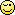27. ### Frustrated Palm

Messages:
4,473
No offense or anything but thats more of a regular hybrid that other people build be cause no regular car (except for exotics) use F1 tires, sounds good though, will try it...

28. ### Frustrated Palm

Messages:
4,473
Working on making the 2003 Integra Type R. The only thing is there isnt any wing or ground effects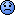...

29. ### F094/H

Messages:
319
Can some one post a pic of a hybrid? I don't know how it looks, or just describe it if it is too much trouble.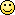30. ### Frustrated Palm

Messages:
4,473
Sometimes there isnt really a difference on the outside like with 'Mild' hybrids. While others will usually have the shine code. Which means they are really really shiney, almost chrome like. Sometimes the wheels stick out of the wheel wells because they are really big or the chassis is longer than normal so the wheels are near the corners or they could even be closer the middle of the car. Usually they are very low as well. Just as low as an F1. Or they can be very high too. It just matters on the hyrbid itself...